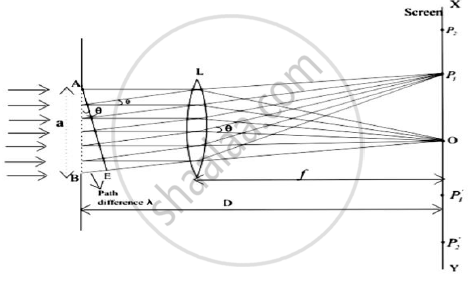# Discuss the Fraunhoffer diffraction at single slit and obtain the condition for minima. - Applied Physics 2

Discuss the Fraunhoffer diffraction at single slit and obtain the condition for minima.
In plane transmission grating the angle of diffraction for second order principal maxima for wavelength 5 x 10-5 is 35o. Calculate number of lines/cm for this diffraction grating.

#### Solution

Fraunhofer diffraction at single slit :

1.Let us first consider a parallel beam of light incident normally on a slit AB of width 'a' which is of order of the wavelength of light.2.A real image of diffraction pattern is formed on the screen with the help of converging lens placed in the path of the diffracted beam

3.All the rays that starts from slit AB in the same phase reinforce each other and produce brightness at point O on the axis of slit as they arrive there in the same phase

4.The intensity of diffracted beam will be different in different directions and there are some directories where there is no light

5.Thus diffraction pattern on screen consists of a central bright band and alternate dark and bright bands of decreasing intensity on both sides

6.Now consider a plane wave front PQ incident on the narrow slit AB. According to Huygens principle each point t on unblocked portion of wavefront PQ sends out secondary wavelets in all directions

7.Their combined effect at any distant point can be found y summing the numerous waves arriving there from the principle of superposition

8.Let C be the center of the slit AB.The secondary waves, from points equidistant from center C of the clit lying on portion CA and CB of wave front travel the same distance in reaching O and hence the path difference between them is zero

9.These waves reinforce each other and give rise to the central maximum at point O
i) Condition for minima :

1.We now consider the intensity at point P1 above O on the screen where another set of rays diffracted at a angle θ have been bought to focus by the lens and contributions from different elements of the slits do not arise in phase at P1

2.If we drop a perpendicular from point A to the diffracted ray from B,then AE as shown in figure constitutes the diffracted wavefront and BE is the path difference between the rays from the two edges A and B of the slit.

3.Let us imagine this path difference to be equal to one wavelength.

4.The wavelets from different parts of the slit do not reach point P1 in the phase because they cover unequal distance in reaching P1.Thus they would interfere and cancel out each other effect. For this to occur

BE = λ

Since BE = ABsinθ

asinθ = λ

or sinθ = λ/a

or θ = λ/a ---(1)

As angle of diffraction is usually very small so that sinθ = θ

5.Such a point on screen as given by the equation (1) would be point of secondary minimum

6.It is because we have assume the slit to be divided into two parts, then wavelets from the corresponding points of the two halves of the slit will have path difference of λ/2  and wavelets from two halves will reach point P1 on the screen in a opposite phase to produce minima

7.Again consider the point P2 in the figure and if for this point path difference BE = 2λ ,then we can imagine slit to be divided into four equal parts

8.The wavelets from the corresponding points of the two adjacent parts of the slit will have a path difference of λ/2 and will mutually interfere to cancel out each other

9.Thus a second minimum occurs at P2 in direction of θ given by θ = (2θ)/a

10.Similarly nth minimum at point Pn occurs in direction of θ given by

θ_n = (nθ)/a  ---(2)

(a+b)sinθ = m λ
(a+b)sin35 = 2 x 5 x 10-5
a+b=1.7434 x 10-4
N = 1/(a+b)

= 5735 ("lines")/(cm)

Number of "lines"/"cm" = 5735

Concept: Diffraction Grating
Is there an error in this question or solution?